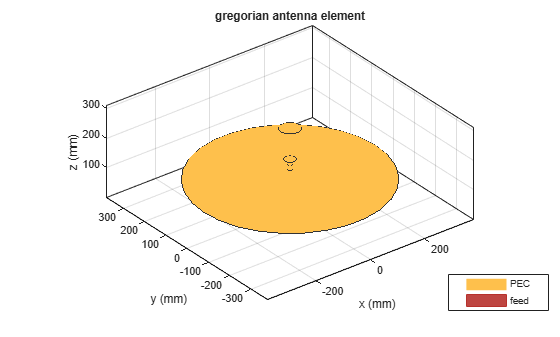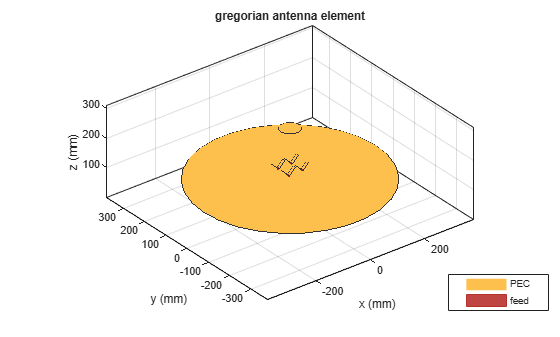Main Content

# gregorian

Create Gregorian antenna

## Description

The `gregorian` object creates a horn conical fed Gregorian antenna. A Gregorian antenna is a parabolic antenna. In this antenna, the feed antenna is mounted at or behind the surface of the main parabolic reflector and aimed at the subreflector. This antenna is used in radio telescopes and communication satellites. For more information see, Architecture of Gregorian Antenna.## Creation

### Syntax

``ant = gregorian``
``ant = gregorian(Name,Value)``

### Description

example

````ant = gregorian` creates a horn conical fed Gregorian antenna with a default operating frequency of 18.48 GHz. This antenna gives maximum gain when operated at 18.3 GHz.```
````ant = gregorian(Name,Value)` sets properties using one or more name-value pairs. For example, ```ant = gregorian('FocalLength',[0.4 0.22])``` creates a Gregorian antenna with the main reflector of focal length 0.4 m and the subreflector of focal length 0.22 m.```

## Properties

expand all

Antenna type used as exciter, specified as an antenna or an array object.

Example: `'Exciter',dipole`

Example: `ant.Exciter = dipole`

Example: ```ant.Exciter = linearArray('patchMicrostrip')```

Radius of the main and subreflector, specified as a two-element vector with each element unit in meters. The first element specifies the radius of the main reflector, and the second element specifies the radius of the subreflector.

Example: `'Radius',[0.4 0.2]`

Example: `ant.Radius = [0.4 0.2]`

Data Types: `double`

Focal length of the main and subreflector, specified as a two-element vector with each element unit in meters. The first element specifies the focal length of the main reflector, and the second element specifies the focal length of the subreflector.

Example: `'FocalLength',[0.35 0.2]`

Example: `ant.FocalLength = [0.35 0.2]`

Data Types: `double`

Lumped elements added to the antenna feed, specified as a lumped element object handle. You can add a load anywhere on the surface of the antenna. By default, the load is at the feed. For more information, see `lumpedElement`.

Example: `'Load',lumpedelement`. `lumpedelement` is the object handle for the load created using `lumpedElement`.

Example: ```ant.Load = lumpedElement('Impedance',75)```

Tilt angle of the antenna, specified as a scalar or vector with each element unit in degrees. For more information, see Rotate Antennas and Arrays.

Example: `'Tilt',90`

Example: `ant.Tilt = 90`

Example: `'Tilt',[90 90]`,`'TiltAxis',[0 1 0;0 1 1]` tilts the antenna at 90 degrees about the two axes defined by the vectors.

Note

The `wireStack` antenna object only accepts the dot method to change its properties.

Data Types: `double`

Tilt axis of the antenna, specified as:

• Three-element vector of Cartesian coordinates in meters. In this case, each coordinate in the vector starts at the origin and lies along the specified points on the X-, Y-, and Z-axes.

• Two points in space, each specified as three-element vectors of Cartesian coordinates. In this case, the antenna rotates around the line joining the two points in space.

• A string input describing simple rotations around one of the principal axes, 'X', 'Y', or 'Z'.

For more information, see Rotate Antennas and Arrays.

Example: `'TiltAxis',[0 1 0]`

Example: `'TiltAxis',[0 0 0;0 1 0]`

Example: `ant.TiltAxis = 'Z'`

Note

The `wireStack` antenna object only accepts the dot method to change its properties.

Data Types: `double`

## Object Functions

 `show` Display antenna or array structure; display shape as filled patch `axialRatio` Axial ratio of antenna `beamwidth` Beamwidth of antenna `charge` Charge distribution on metal or dielectric antenna or array surface `current` Current distribution on metal or dielectric antenna or array surface `design` Design prototype antenna or arrays for resonance at specified frequency `EHfields` Electric and magnetic fields of antennas; Embedded electric and magnetic fields of antenna element in arrays `impedance` Input impedance of antenna; scan impedance of array `mesh` Mesh properties of metal or dielectric antenna or array structure `meshconfig` Change mesh mode of antenna structure `optimize` Optimize antenna or array using SADEA optimizer `pattern` Radiation pattern and phase of antenna or array; Embedded pattern of antenna element in array `patternAzimuth` Azimuth pattern of antenna or array `patternElevation` Elevation pattern of antenna or array `returnLoss` Return loss of antenna; scan return loss of array `sparameters` S-parameter object `vswr` Voltage standing wave ratio of antenna

## Examples

collapse all

Create and view a default Gregorian antenna.

`ant = gregorian`
```ant = gregorian with properties: Exciter: [1×1 hornConical] Radius: [0.3175 0.0330] FocalLength: [0.2536 0.1416] Tilt: 0 TiltAxis: [1 0 0] Load: [1×1 lumpedElement] ```
`show(ant)`Plot the radiation pattern of the antenna at 18.48 GHz.

`pattern(ant,18.48e9)`Create an array of vee dipole antennas.

```e = dipoleVee('ArmLength',[0.03 0.03],'Width',0.01); arr = rectangularArray('Element',e,'RowSpacing',0.05,'ColumnSpacing',0.05);```

Create a Gregorian antenna with rectangular array as exciter

```ant = gregorian('Exciter',arr); show(ant)```expand all

## See Also

### Topics

Introduced in R2019b

## Support

#### Hybrid Beamforming for Massive MIMO Phased Array Systems

Download the white paper